# NCERT Solutions for Class 10 Maths Chapter 5 Arithmetic Progressions Ex 5.2

Get Free NCERT Solutions for Class 10 Maths Chapter 5 Ex 5.2 PDF. Arithmetic Progressions Class 10 Maths NCERT Solutions are extremely helpful while doing your homework. Exercise 5.2 Class 10 Maths NCERT Solutions were prepared by Experienced LearnCBSE.in Teachers. Detailed answers of all the questions in Chapter 5 Maths Class 10 Arithmetic Progressions Exercise 5.2 provided in NCERT TextBook.

Topics and Sub Topics in Class 10 Maths Chapter 5 Arithmetic Progressions:

 Section Name Topic Name 5 Arithmetic Progressions 5.1 Introduction 5.2 Arithmetic Progressions 5.3 Nth Term Of An AP 5.4 Sum Of First N Terms Of An AP 5.5 Summary

You can also download the free PDF of  Ex 5.2 Class 10 Arithmetic Progressions NCERT Solutions or save the solution images and take the print out to keep it handy for your exam preparation.

 Board CBSE Textbook NCERT Class Class 10 Subject Maths Chapter 5 Chapter Name Arithmetic Progressions Exercise Ex 5.2 Number of Questions Solved 20 Category NCERT Solutions

## NCERT Solutions for Class 10 Maths Chapter 5 Arithmetic Progressions Ex 5.2

NCERT Solutions for Class 10 Maths Chapter 5 Arithmetic Progressions Ex 5.2 are part of NCERT Solutions for Class 10 Maths. Here we have given NCERT Solutions for Class 10 Maths Chapter 5 Arithmetic Progressions Exercise 5.2

Question 1.
Fill in the blanks in the following table, given that a is the first term, d the common difference and the nth term of the AP:Solution: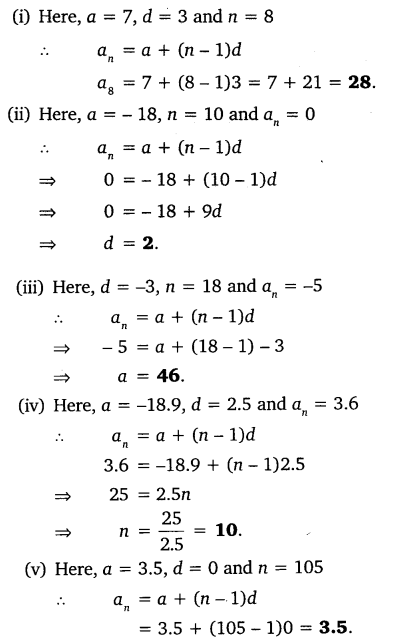Question 2.
Choose the correct choice in the following and justify:
(i) 30th term of the AP: 10, 7, 4, …, is
(a) 97
(b) 77
(c) -77
(d) -87

(ii) 11th term of the AP: -3,$\frac { -1 }{ 2 }$, 2, …, is
(a) 28
(b) 22
(c) -38
(d) -48
Solution:Question 3.
In the following APs, find the missing terms in the boxes:Solution: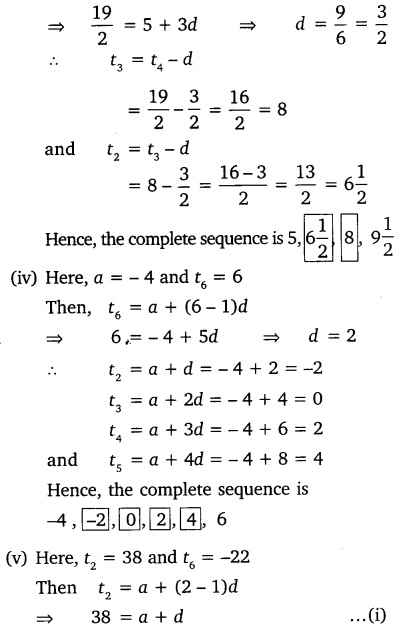Question 4.
Which term of the AP: 3, 8, 13, 18, …, is 78?
Solution:Question 5.
Find the number of terms in each of the following APs:
(i) 7, 13, 19, …, 205
(ii) 18, 15$\frac { 1 }{ 2 }$, 13, …, -47
Solution:Question 6.
Check, whether -150 is a term of the AP: 11, 8, 5, 2, ….
Solution: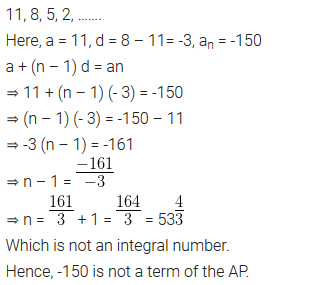Question 7.
Find the 31st term of an AP whose 11th term is 38 and the 16th term is 73.
Solution:Question 8.
An AP consists of 50 terms of which 3rd term is 12 and the last term is 106. Find the 29th term.
Solution:Question 9.
If the 3rd and the 9th term of an AP are 4 and -8 respectively, which term of this AP is zero?
Solution:Question 10.
The 17th term of an AP exceeds its 10th term by 7. Find the common difference.
Solution:Question 11.
Which term of the AP: 3, 15, 27, 39, … will be 132 more than its 54th term?
Solution:Question 12.
Two APs have the same common difference. The difference between their 100th terms is 100, what is the difference between their 1000th terms?
Solution:Question 13.
How many three-digit numbers are divisible by 7?
Solution:Question 14.
How many multiples of 4 lie between 10 and 250?
Solution:Question 15.
For what value of n, the nth term of two APs: 63, 65, 61,… and 3, 10, 17,… are equal?
Solution:Question 16.
Determine the AP whose 3rd term is 16 and 7th term exceeds the 5th term by 12.
Solution:Question 17.
Find the 20th term from the last term of the AP: 3, 8, 13, …, 253.
Solution: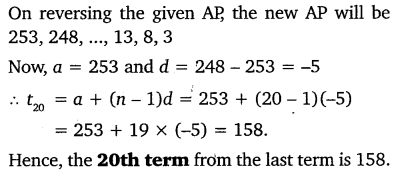Question 18.
The sum of the 4th and 8th terms of an AP is 24 and the sum of the 6th and 10th terms is 44. Find the first three terms of the AP.
Solution: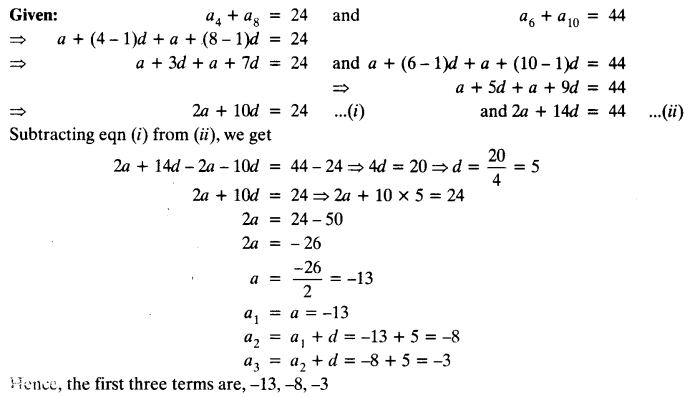Question 19.
Subba Rao started work in 1995 at an annual salary of ₹ 5000 and received an increment of ₹ 200 each year. In which year did his income reach ₹ 7000 ?
Solution: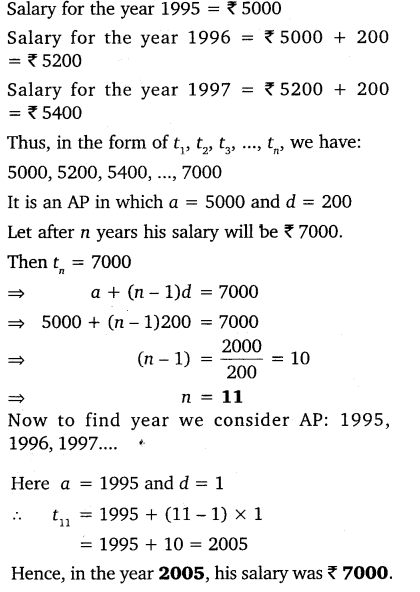Question 20.
Ramkali saved ₹ 5 in the first week of a year and then increased her weekly saving by ₹ 1.75. If in the nth week, her weekly saving become ₹ 20.75, find n.
Solution:+